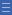## Featured resource

Home > Topdrawer > Fractions > Good teaching > Dividing > Fractions of collections

# Fractions of collections

Fractions of collections

Finding a fraction of a collection of items is actually finding a fraction of a whole number. The process involves making equal groups and is therefore the same as the sharing-as-division process.

Finding one-third of a number is the same as dividing by three, and can be represented with materials in a similar way.

For example, $$\frac{1}{3}$$ of 12 and 12 ÷ 3 could both be modelled using 12 counters partitioned into three equal groups of four.

The mathematical relations suggested by the three equal groups of four counters are:

 3 $$\times$$ 4 = 12 12 ÷ 3 = 4 $$\frac{1}{3}$$ of 12 = 4

Working with fractions of collections is helped by a sound knowledge of factors and multiples.

Using arrays and area grids strengthens the relationships between multiplication, division and fractions, by making the inverse relations more apparent.

 3 $$\times$$ 4 = 12 4 $$\times$$ 3 = 12 12 ÷ 3 = 4 12 ÷ 4 = 3 $$\frac{1}{3}$$ of 12 = 4 $$\frac{1}{4}$$ of 12 = 3

Fractions also appear in whole-number division when remainders occur.

For example, $$\frac{1}{3}$$ of 13 (or 13 ÷ 3) results in 4 remainder 1. The remainder 1 can be partitioned into three equal parts and the sharing process continued, leading to the mixed-number answer $$4\frac{1}{3}$$.

Yes

Yes

Name Class SectionPart and wholes Folder 17Divide it up Folder 17Year 2: Recognise and interpret common uses of halves, quarters and eighths of shapes and collections Infobox 3Year 6: Find a simple fraction of a quantity where the result is a whole number, with and without digital technologies Infobox 3Source Infobox 3